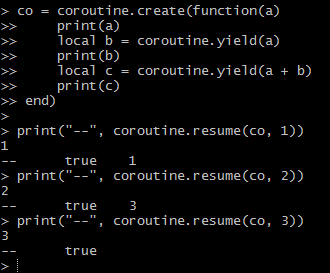# 使用协程处理耗时过程

function deal(list)
local p = 0
local function foo()
local from, to = p + 1, math.min(p + 50, #list)
for i = from, to do
local id = list[i]
dosth(id)
end
p = to
if p < #list then
timer:start_once(1, foo)
end
end
foo()
endcounts = {}
max_counts = {}
function try_yield()
local co = coroutine.running()
assert(co ~= nil)
counts[co] = counts[co] + 1
if counts[co] >= max_counts[co] then
counts[co] = 0
coroutine.yield()
end
end

function with_coroutine(f, n, t)
return function(...)
local co = coroutine.create(f)
counts[co] = 0
max_counts[co] = n

local function foo(...)
coroutine.resume(co, ...)
counts[co] = nil
max_counts[co] = nil
return
end
timer:start_once(t, foo)
end
foo(...)
end
end


deal = with_coroutine(function(list)
for i, id in ipairs(list) do
dosth(id)
try_yield()
end
end, 50, 1)